Question

# Peavey Enterprises purchased a depreciable asset for \$32,000 on April 1, Year 1. The asset will...

Peavey Enterprises purchased a depreciable asset for \$32,000 on April 1, Year 1. The asset will be depreciated using the straight-line method over its four-year useful life. Assuming the asset's salvage value is \$4,000, what will be the amount of accumulated depreciation on this asset on December 31, Year 3?

Multiple Choice

\$19,250

\$5,833

\$5,833

\$7,000

\$23,333

Solution:

Annual Depreciation = (cost - salvage) / 4 = (\$32000 - \$4000) / 4 = \$7,000

Depreciation for Year 1 (for 9 months) = \$7000*9/12 = \$5,250

Accumulated Depreciation on December 31, Year 3 = \$5250 + \$7000 + \$7000 = \$19,250

Hence first option is correct.

#### Earn Coins

Coins can be redeemed for fabulous gifts.

Similar Homework Help Questions
• ### Peavey Enterprises purchased a depreciable asset for \$22,000 on April 1, Year 1. The asset will be depreciated using the...

Peavey Enterprises purchased a depreciable asset for \$22,000 on April 1, Year 1. The asset will be depreciated using the straight-line method over its four-year useful life. Assuming the asset's salvage value is \$2,000, Peavey Enterprises should recognize depreciation expense in Year 2 in the amount of:A) \$10,000B) \$5,000C) \$5,500D) \$20,000E) \$9,250

• ### Lima Enterprises purchased a depreciable asset for \$31,500 on April 1, Year 1. The asset will...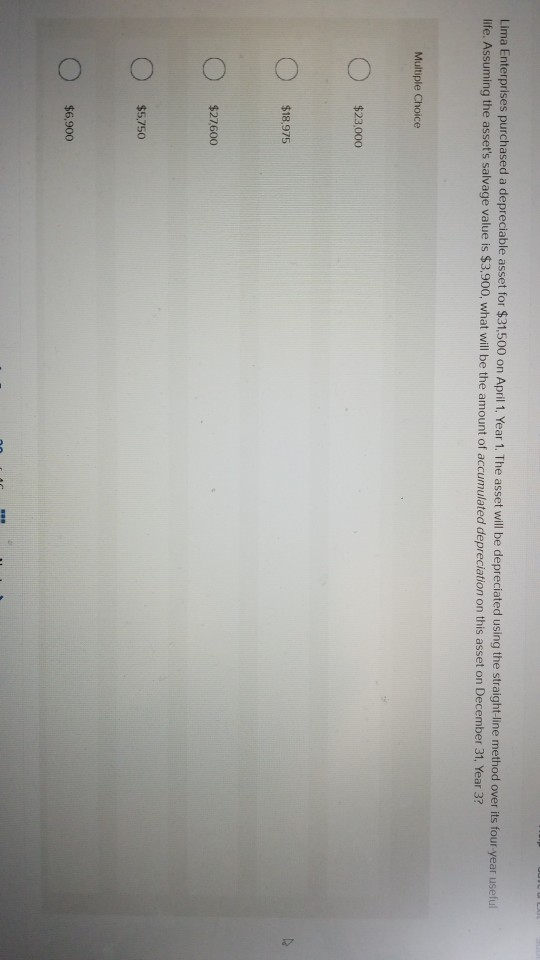Lima Enterprises purchased a depreciable asset for \$31,500 on April 1, Year 1. The asset will be depreciated using the straight-line method over its four year useful life. Assuming the asset's salvage value is \$3,900, what will be the amount of accumulated depreciation on this asset on December 31, Year 3? Multiple Choice Ο \$23,000 Ο \$18,975 Ο \$27600 Ο \$5.750 Ο \$6,900

• ### (5 points) Peavey Enterprises purchased a depreciable asset for \$22,000 on April 1. Year 1. The...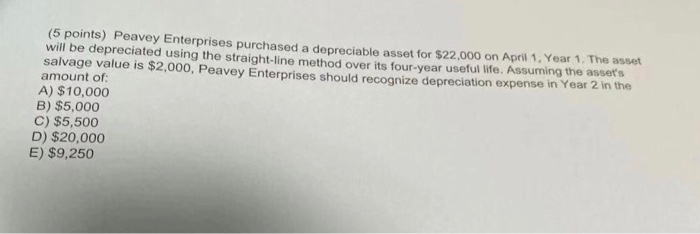(5 points) Peavey Enterprises purchased a depreciable asset for \$22,000 on April 1. Year 1. The asset will be depreciated using the straight-line method over its four-year useful life. Assuming the assets salvage value is \$2,000, Peavey Enterprises should recognize depreciation expense in Year 2 in the amount of: A) \$10,000 B) \$5,000 C) \$5,500 D) \$20,000 E) \$9,250

• ### Peavey Enterprises purchased a depreciable asset for \$31000 on April1, Year 1. The asset will be...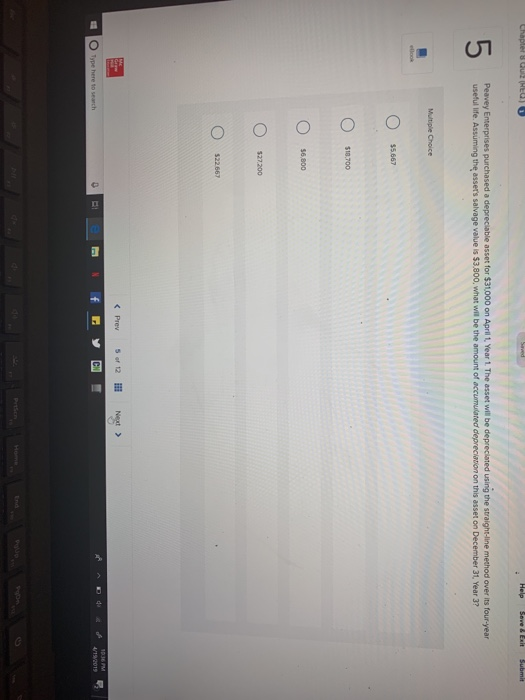Peavey Enterprises purchased a depreciable asset for \$31000 on April1, Year 1. The asset will be depreciated using the straight-line method over its four-year useful life. Assuming the assets salvage value is \$3.800, what will be the amount of accumuliated depreciation on this asset on December 31, Year 3? \$18.700 6,800 \$22667 Type here to search /192019

• ### Thomas Enterprises purchased a depreciable asset on October 1. Year 1 at a cost of \$100,000....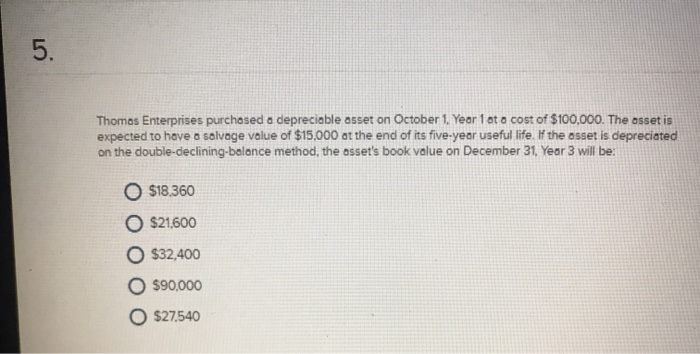Thomas Enterprises purchased a depreciable asset on October 1. Year 1 at a cost of \$100,000. The asset is expected to have a salvage value of \$15,000 at the end of its five-year useful life. If the asset is depreciated on the double-declining balance method, the asset's book value on December 31, Year 3 will be: \$18.360 O \$21,600 \$32,400 \$90,000 \$27.540 Lomax Enterprises purchased a depreciable asset for \$22.500 on March 1, Year 1 The asset will be depreciated...

• ### Beckman Enterprises purchased a depreciable asset on October 1. Year 1 at a cost of \$100.000....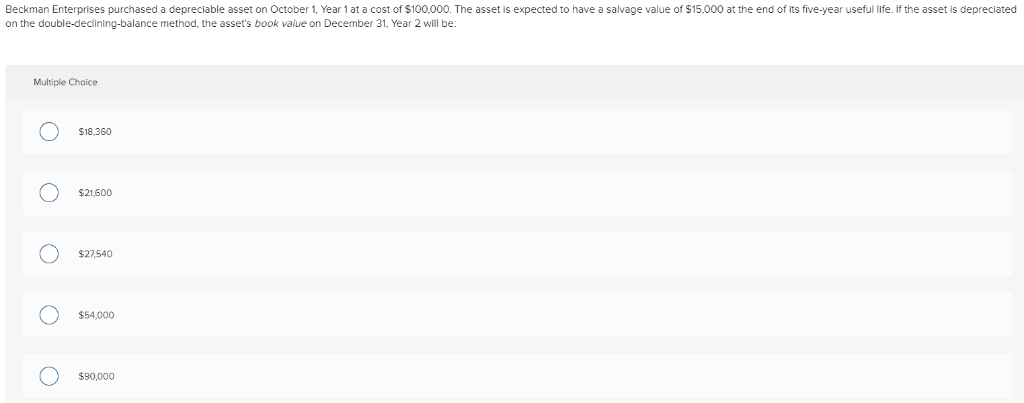Beckman Enterprises purchased a depreciable asset on October 1. Year 1 at a cost of \$100.000. The asset is expected to have a salvage value of \$15.000 at the end of its five-year useful life. If the asset is depreciated on the double-declining-balance method, the asset's book value on December 31. Year 2 will be: Multiple Choice \$18,360 \$21,600 \$27540 \$54,000 \$90,000

• ### Lima enterprises purchased a depreciable asset for 27500 on April 1 , year 1. The asset will be depreciated using the st...

Lima enterprises purchased a depreciable asset for 27500 on April 1 , year 1. The asset will be depreciated using the striaght line method over it 4 year useful life. Assuming the assets salvage value is 3100, Lima enterprises should recognize depreciation expense in year 2 in the amount of: A. 23,383.33 B. 5083.33 c. 6100.00 d. 24,400.00 e. 6875.00

• ### Colvin Enterprises purchased a depreciable asset on October 1, Year 1 at a cost of \$148,000....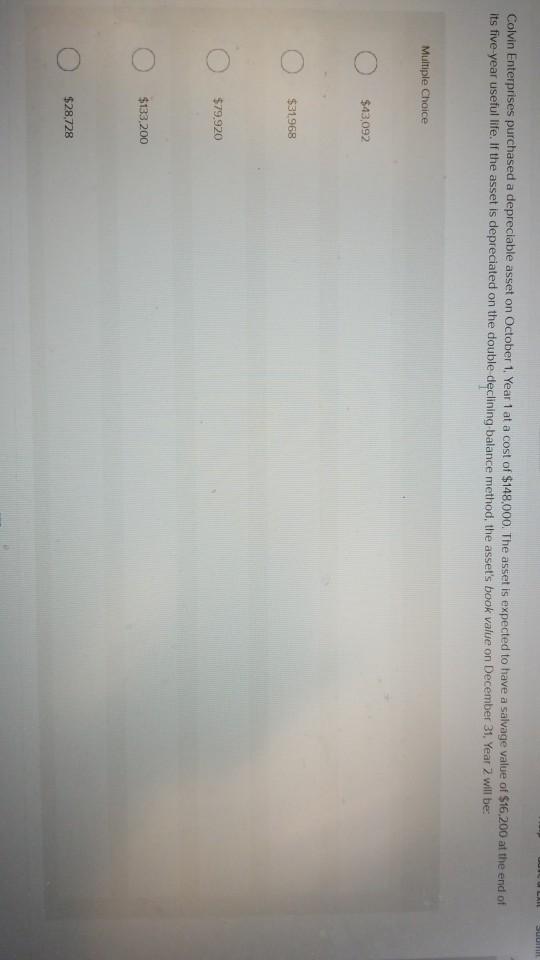Colvin Enterprises purchased a depreciable asset on October 1, Year 1 at a cost of \$148,000. The asset is expected to have a salvage value of \$16,200 at the end of its five year useful life. If the asset is depreciated on the double declining balance method, the asset's book value on December 31, Year 2 will be Multiple Choice O \$43,092 O \$31968 \$79.920 \$133 200 \$28,728

• ### Colvin Enterprises purchased a depreciable asset on October 1. Year 1 at a cost of \$116,000....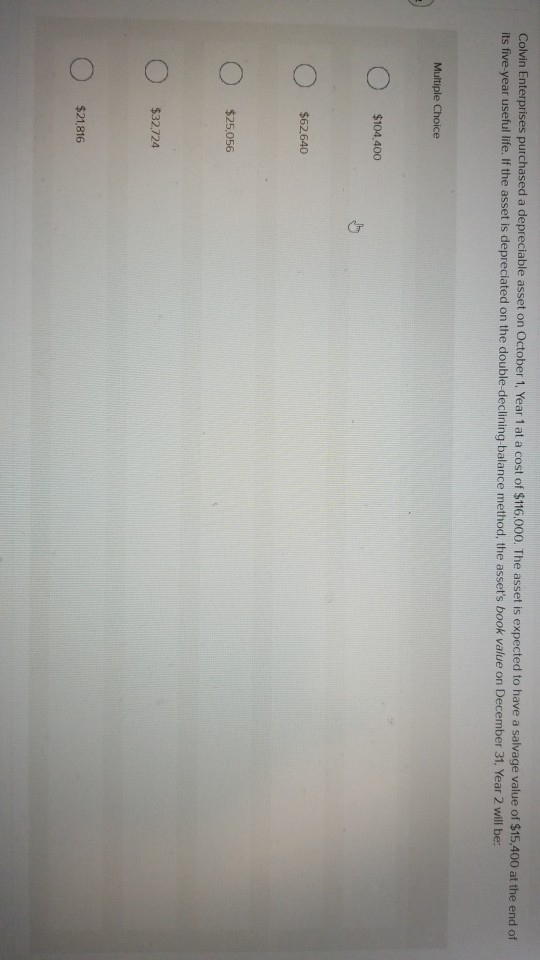Colvin Enterprises purchased a depreciable asset on October 1. Year 1 at a cost of \$116,000. The asset is expected to have a salvage value of \$15,400 at the end of its five year useful life. If the asset is depreciated on the double-declining balance method, the asset's book value on December 31, Year 2 will be: Multiple Choice Ο \$104,400 Ο \$62640 Ο \$25,056 Ο \$32724 Ο \$21,816

• ### 28) When originally purchased, a vehicle costing \$26.460 had an estimated useful life of 8 years...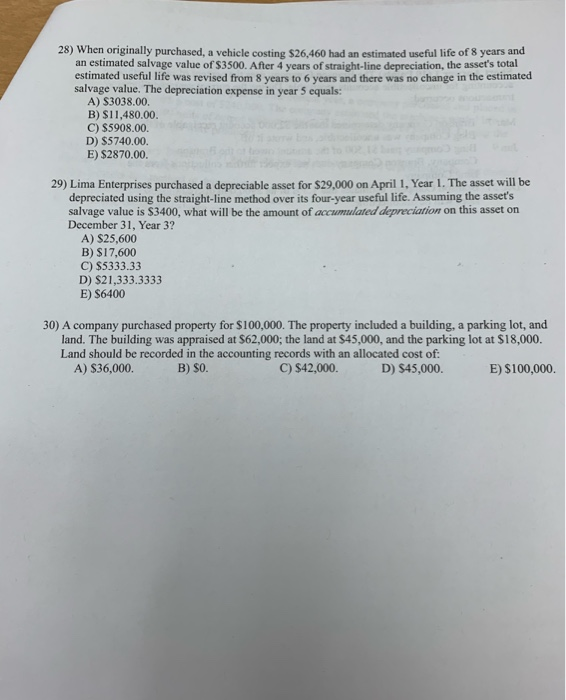28) When originally purchased, a vehicle costing \$26.460 had an estimated useful life of 8 years and an estimated salvage value of \$3500. After 4 years of straight-line depreciation, the asset's total estimated useful life was revised from 8 years to 6 years and there was no change in the estimated salvage value. The depreciation expense in year 5 equals: A) S3038.00. B) \$11,480.00. C) \$5908.00. D) \$5740.00 E) \$2870.00. 29) Lima Enterprises purchased a depreciable asset for \$29.000 on...